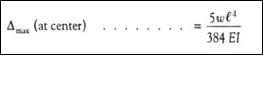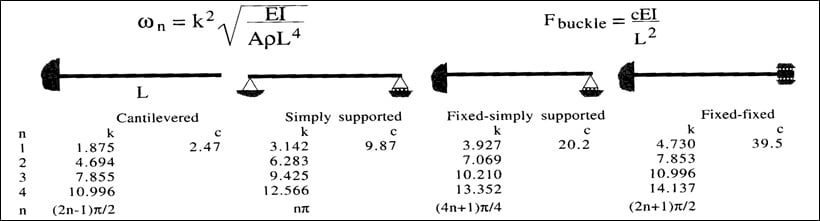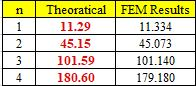## General Mechanical

•smehboob
Subscriber

I am facing a problem in modal analysis results. A simply supported steel beam of dimensions as Length = 4000 mm, Cross section = 250 x 150 mm, E=2E+11 N/m^2, Density = 7850 kg/m^3. Please note, beam is along X-Axis.

There is a difference of theoretical and FEM results. First two bending natural frequencies are almost comparable as you can see below while in higher modes like 3rd and 4th there is significant difference in results.

I am confused, how it would be done, so that i can get all FE results according to theoretical values?

Looking forward for the solution if someone could help in this matter.

Thanks

•peteroznewman
Subscriber

You give more detail about the Boundary Conditions in this post where you said the right end has a displacement of Y=0.  That is not what I would call a simply supported beam.

In any case, I don't get the frequencies you are showing.  Please attach a .wbpz Archive file for further review.

•smehboob
Subscriber

a

Dear Peteroznewman

Note: This is my second trial beam analysis, i had provided the BCs as fixed in a way you can see in .wbpz file. Then compared the calculated the max. mid span deflection and the 1st four bending natural frequencies.

In this case probably the depth is relatively less as compared to its length, thus yielding the reasonable good FEM results with negligible error difference.Above equation gives Delta Max. = 0.00247 m

Theoretical, Natural frequencies are calculated using the relation shown below:Now the FEM results are as follows:Can you please comment on the applied BCs and the approach i am following, is it correct?

Thank in anticipation

•smehboob
Subscriber
•Erik Kostson
Ansys Employee
•smehboob
Subscriber

Ekoston. Thank you for your help. I have just picked my mistake.